﻿ Generation Maintenance Scheduling for Generation Expansion Planning Using a Multi-Objective Binary Gravitational Search Algorithm

### Generation Maintenance Scheduling for Generation Expansion Planning Using a Multi-Objective Binary G...OPEN ACCESSPEER-REVIEWED

## Generation Maintenance Scheduling for Generation Expansion Planning Using a Multi-Objective Binary Gravitational Search Algorithm

Iman Goroohi Sardou1,, Mohammad Taghi Ameli1, Mehrdad Setayesh nazar1

1Faculty of Electrical and Computer Engineering, Shahid Beheshti University, Tehran, Iran

### Abstract

Generation maintenance scheduling (GMS) is an important and effective part of Generation expansion planning (GEP) problem. Preventive-maintenance schedules need to be optimized to trade-off between two conﬂicting objectives, reducing the overall cost and improving the reliability. This paper presents a multi-objective binary gravitational search algorithm (BGSA) for solving GMS problem of generation systems as a sub-problem of the main GEP problem. In the proposed method, a fuzzy membership function is defined for each term in the objective function. There are three objective functions in this problem. The first objective function is leveling reserve capacity when unit maintenance outages are considered. The second and the third objectives which are also objectives of the main GEP problem, are to minimize the operation and maintenance (O&M) cost and the reliability index of Expected Energy Not Supplied (EENS). As GMS problem is a sub-problem of the main GEP problem, it is solved for a typical solution of the main GEP problem. The proposed method is applied to solve GMS problem for 4-bus test system from Grainger & Stevenson and IEEE-RTS 24-bus test system for a planning horizon of one year and two years, respectively. To verify the capability of the proposed BGSA based method, a binary genetic algorithm (BGA) method is also implemented to solve GMS problem and then the results are compared.

### At a glance: Figures

123
Prev Next

• Sardou, Iman Goroohi, Mohammad Taghi Ameli, and Mehrdad Setayesh nazar. "Generation Maintenance Scheduling for Generation Expansion Planning Using a Multi-Objective Binary Gravitational Search Algorithm." International Transaction of Electrical and Computer Engineers System 2.2 (2014): 44-55.
• Sardou, I. G. , Ameli, M. T. , & nazar, M. S. (2014). Generation Maintenance Scheduling for Generation Expansion Planning Using a Multi-Objective Binary Gravitational Search Algorithm. International Transaction of Electrical and Computer Engineers System, 2(2), 44-55.
• Sardou, Iman Goroohi, Mohammad Taghi Ameli, and Mehrdad Setayesh nazar. "Generation Maintenance Scheduling for Generation Expansion Planning Using a Multi-Objective Binary Gravitational Search Algorithm." International Transaction of Electrical and Computer Engineers System 2, no. 2 (2014): 44-55.

 Import into BibTeX Import into EndNote Import into RefMan Import into RefWorks

### 1. Introduction

Generation Expansion Planning (GEP) is the ﬁrst key step in long-term planning issues, after correctly forecasting the load for a speciﬁed future period. In general terms, GEP is an optimization problem in which the aim is to determine the new generation plants in terms of when to be invested, what type and capacity they should be and where they should be installed, so that the cost function is minimized and various constraints are satisfied. An essential feature means that the reliability requirement within the power industry is very high. One of the main subjects that is of great importance to the reliability of power systems is to maintain the availability of generating units in an acceptable level. On the other hand, the maintenance schedules of power generating units play a critical role in both planning and operation management sections of power system. The number of generators is increased and the number of reserve margins are decreased by the electricity demand growing in modern power systems. Consequently, GMS has become a complex, multi-object-constrained optimization problem for power systems [1, 2, 3, 4]. unit commitment, generation dispatch, import/export of generating units, generation expansion planning and such other planning activities are directly affected by GMS problem decisions. For this reason, GMS problem is essential to solve for the planning of the certain and reliable power system operation in a modern power system. The purpose of maintenance scheduling is to ﬁnd the sequence of scheduled outages of generating units over a given period of time such that the level of energy reserve is maintained. A lot of research has been carried on GMS for several decades. A fuzzy system approach with genetic enhancement is applied to GMS in . In this reference, fuzzy sets are used to optimally adjust membership functions parameters. [2-7] have introduced meta-heuristic algorithms based approaches such as modiﬁed discrete particle swarm optimization (MDPSO) and simulated annealing to form robust methods for solving the GMS problem. In these references, economic beneﬁts and reliable operation of a power system are improved, subject to satisfying system load demand, allowable maintenance window, crew and resource constraints. Security-constrained GMS problem are solved in [8, 9, 10, 11] using an evolutionary programming-based solution technique and general algebraic modeling system, considering uncertainties in the load, fuel and maintenance costs. In [12, 13], formulations are presented that enable Ant Colony Optimization (ACO) for continuous domains to seek the optimal solution of GMS problem. The objective functions of these formulations consider the effect of economy as well as reliability. In these references, various constraints such as spinning reserve, duration of maintenance crew are taken into account. [14, 15, 16] have proposed a decision tree and mixed integer linear formation for solving the GMS problem that clearly consider the thermal units aging momentum. This allows the system operators to determine the thermal units’ maintenance outage schedule based on the cost analysis of maintenance tasks. This cost analyzing should be done by optimizing the time interval between consecutive maintenance tasks.

In the present paper, GMS problem is solved as a sub-problem of the main GEP problem to optimize and readjust the maintenance scheme of generating units according to the economical indices and reliability indices of the system operation in different times. A multi-objective fuzzy approach is used to solve GMS problem. Two objectives of minimizing O&M cost and minimizing the EENS index are common to the GMS and GEP problems. Therefore, a probabilistic production simulation is proposed to obtain EENS index and O&M cost of the generating units according to their maintenance schedules. The risk of the system's supply being not sufficient may be increased during the scheduled maintenance outages. Therefore, leveling reserve capacity (the system's installed capacity minus the maximum load and the maintenance capacity during the period under examination) is considered as the another objective function to guarantee the electricity supply reliability of the power system.

Gravitational Search Algorithm (GSA) [17, 18] is based on the law of gravity and mass interactions. The higher efficiency of GSA in compared to some other heuristic optimization methods such as Particle Swarm Optimization (PSO), Central Force Optimization (CFO) and Real Genetic Algorithm (RGA) in solving various nonlinear functions, are demonstrated in .  has examined GSA on a set of various standard bench mark functions. The results obtained by GSA in most cases have provided superior results and in all cases were comparable with PSO, RGA and CFO. Consequently, Binary Gravitational Search Algorithm (BGSA) is used as the optimization tool to solve GMS problem in the present paper. Besides, a fuzzy membership function is defined for each term in the objective function. GMS problem is solved for the best solution of the main GEP problem that is evaluated without considering maintenance scheduling of generating units. 4-bus test system from Grainger & Stevenson for a planning horizon of one year and IEEE-RTS 24-bus test system for a planning horizon of two years are used as test systems. BGA is also implemented to solve GMS problem to satisfy the effectiveness of the proposed BGSA, and then the results are compared and interpreted. The results indicated that the BGSA is an effective alternative for the solution of the proposed GMS problem. The main contribution of this paper is summarized at the following:

-Proposing a new methodology for solving GMS problem as a sub-problem of the main GEP problem taking into account the economical indices and reliability indices

-Proposing new formulation for computing O&M cost and EENS

-Applying multi-objective BGSA to solve the proposed GMS problem and verifying its compatibility in comparison with BGA.

### 2. Problem Formulation

In this section, conﬁgured formulation for GMS problem has been developed. The problem is to determine the set of generating units involving in maintenance in each time interval. In fact, GMS variables are time intervals and day number of them, when each generating unit is allowed to be scheduled for maintenance. In doing so, besides satisfying various constraints, such as the maintenance crew constraint and transmission state constraint, the present value of O&M costs and the index of EENS should be minimized. In addition the reserve capacity is levelized during the time intervals. The proposed objective functions as well as the various constraints are discussed at the following subsections.

2.1. Fuzzy Multi-objective Formulation

A fuzzy optimization procedure is used in this paper for handling the multiple objectives. In this approach, objective function terms can be summed up by transforming elements of objective functions into fuzzy domain with a suitable membership function. Membership functions indicate the degree of satisfaction of the objectives. A higher membership value implies greater satisfaction with the solution. The shape of membership function depends on the nature of the related objective and it may be decided by the decision maker [19, 20, 21]. Overall satisfaction of plan is obtained from Eq. (1).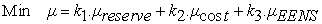(1)

Where, k1, k2, k3 are the non-negative weighting coefficients satisfying the condition: k1+k2+k3=1, which can be tuned using the expert knowledge. Depending on the purpose, the optimization procedure can be readily adapted simply by changing the values of the weighting coefficients and this is an interesting feature for the multi-objective formulation.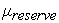,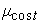andare respectively the membership function values for three objective functions, levelizing the reserve capacity, minimizing O&M cost and minimizing the reliability index of EENS, which are described at the following.

In the crisp domain, either the objective is satisfied or it is violated, respectively, membership values of unity or zero are implied. Fuzzy set membership may have a value from zero to unity. Lower and upper bound values of an objective with a strictly monotonically decreasing and continuous function, create a membership function. A commonly used membership function is used for both objective functions employed in this problem, as shown in Figure 1 [21, 22]. This relationship is expressed mathematically in Eq. (2). Index ofin this figure and equation can be replaced by indices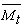, EENS and Iresv.(2)

As can be seen from Figure 1, a solution with a lower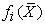has a greater membership value, and vice versa. In addition,andcan be calculated by solving the single objective problem for minimizing and maximizing the objective function, separately .

Figure 1. Membership function used for all objective functions () can be replaced by any of indices Mt, EENS or Iresv)
2.2. Levelized Reserve Capacity

For convenience in scheduling, the whole maintenance period is divided into Nst stages that each stage is either one week, ten days or one month. The levelized reserve method is a straight and instinctive method that draws up a maintenance schedule by leveling the system's net reserve capacity throughout the time intervals. Actually, sum of the system load and capacity of generating unit's maintenance outages throughout the time subintervals are made uniform in this method. Not that, the time subintervals are specified during the time intervals, considering the starting day and the length of generating units maintenance outages. This is a method conventionally used in GMS problem and it has found wide application in electric utilities owing to its easy and understandable concept. As aforementioned, reserve capacity leveling is one of the objective function of the proposed method. In doing so, an index is defined to evaluate this objective function. Figure 2 and Eq. (3) are used to calculate this index, taking into account the generating unit's maintenance outages.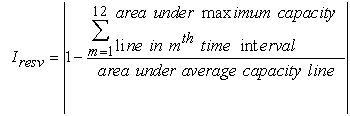(3)

As can be seen,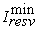is equal to 0, as long as the maximum capacity is the same throughout the time intervals. Nint is total number of time subintervals, that is dependent on GMS problem solution.

2.3. O&M Cost

O&M cost is one of the objectives used in proposed fuzzy multi-objective approach. O&M costs in each year are composed of fuel costs and non-fuel O&M costs of generation, which can be evaluated as:(4)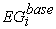,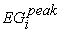and,respectively, denote the energy generated in base and peak capacity, and total energy generated by the type-k ith plant unit located at bus b, in period f of year t of the study period. In this study, the energy generated by each unit in the system is calculated by probabilistic production simulation. In this approach the forced outages of thermal units are convolved with the inverted equivalent load duration curve (ELDC) and, consequently, the effect of unexpected outages of thermal units upon other units is accounted in a probabilistic way. The net effect is that the expected generating costs of the system is increased by an increase of peaking units generation in order to make up the reduction of base units generation due to scheduled outages for maintenance and unit failures . Indices of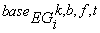,and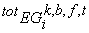are calculated using probabilistic production simulation considering an ELDC. Eq. (5) shows the equation of the ELDC for fixed and candidate units:(5)

Where Pi is the operation rate of generating unit i and qi=1-pi is equal to the unit’s Forced Outage Rate (FOR);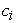is generating capacity for unit i in p.u; energy generated by each unit is determined using Eq. (6)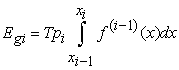(6)

Where xi is equal to.

2.4. Expected Energy not Supplied

As already mentioned, EENS is another objective function of the proposed method. A generation unit maybe tripped at a rate given by its FOR, which represents the percentage of time, when unit maybe unavailable due to unexpected outages. Some portion of the energy demand may not be served owing to generating unit's FORs and maintenance outages. Probabilistic production simulation involving ELDC is also implemented to compute EENS as in Eq. (7).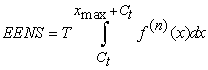(7)

whereis the total capacity of all of the generating units during the time intervals.

2.5. Constraints of the Proposed Method

In the proposed GMS problem, two types of constraints are imposed: technical constraints comprising of GEP constraints and basic constraints consisting of fuel constraints, maintenance constraints, maintenance crew constraints and transmission state constraints. The transmission state constraints are some special limits imposed on the generating unit maintenance plan in a certain area by the transmission network operation state. Some of these constraints are described at the following:

2.5.1. Technical Constraints

Generation capacity should be sufficient in meeting the load requirements, though there are uncertainties that may cause generation units to trip unexpectedly at any time as in Eq. (8). Eq. (8) simply states that the installed capacity in the critical period must lie between the given maximum and minimum reserve margins—at and bt, respectively—above the peak demand (Dt,c) during the critical period of the year.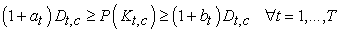(8)

2.5.2. Basic Constraints

a) The continuity of maintenance activity: Maintenance must be completed in a continuous time interval once started as in Eq. (9)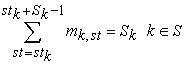(9)

b) The minimum time interval between two maintenances to each generating unit: Suppose that the time interval is considered as N stages; then this constraint for unit k is presented as follows: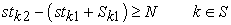(10)

c) Maintenance crew constraint: The maintenance crew constraint means that there are limitations due to the maintenance manpower availability; Normally, two generating units cannot be scheduled for maintenance together in the same power plant and at the same time, i.e. Vr,st=1. Only a few power plants with considerable maintenance resources can allow Vr,st>1. However, the following equation must still be met :(11)

### 3. Solution Methodology

As aforementioned, the purpose of this problem is to find the optimum maintenance schedule of generating units. The objectives are described in Section 2. In this section, firstly, BGSA is described, and then it is employed to the proposed method.

3.1. Binary Gravitational Search Algorithm (BGSA)

In the BGSA [17, 18], agents are considered as objects and their performance is measured by their masses. All these objects attract each other by the gravity force, and a global movement of all objects towards the objects with heavier masses, is generated by this force. As a result, masses cooperate using a direct form of communication, through gravitational force. The heavy masses– which correspond to good solutions – guarantees the exploitation step of the algorithm, owing to their more slowly movement than lighter ones. The BGSA could be considered as a small artificial world of masses obeying the Newtonian laws of gravitation and motion system of masses. The flow diagram of BGSA is depicted in Figure 3. worst(t) and best(t) in this figure are defined as follows (for a minimization problem):(12)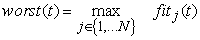(13)

Where, fiti(t) represent the fitness value of the agent i at time t. In BGSA a system with N agents (masses) is considered. The position of the ith agent is defined by: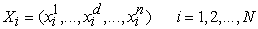(14)

The step of Fitness evaluation is performed using following equations:(15)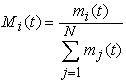(16)

To update agents’ position Eqs. (17-24) are used: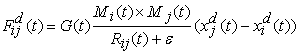(17)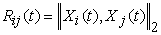(18)

The total force that acts on agent i in a dimension d () is supposed to be a randomly weighted sum of dth components of the forces exerted from other agents, giving a stochastic characteristic to the algorithm: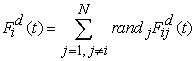(19)

Where r and j is a random number in the interval [0,1][, 1].(20)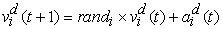(21)is limited to an appropriate interval for a better convergence as Eq. (22).(22)

In BGSA a probability function is defined for each agent as: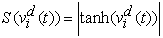(23)

Position change of each agent in a dimension of binary space will cause its value change from 0 to 1 or vice versa. Following the above calculation of probability function each agent moves in each dimension as Eq. (24).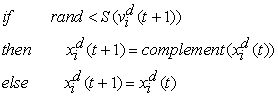(24)

#### Table 1. Integer structure and corresponding description for the position of each agent in each dimension

3.2. Applying BGSA to the Proposed Method

To solve GMS problem using BGSA, problem variables are combined and represented as a mixed integer coding in each agent position. The data structure of each agent position is depicted in Figure 4. As can be seen from this figure, the binary to decimal conversion of each five bits of position of each agent in each dimension refers to the starting day of the time stage st when generating unit u is allowed to be scheduled for maintenance in year t of the study period, as shown in Table 1. In the proposed BGSA, candidate solutions of initial population are randomly selected between all solutions which satisfy the problem constraints and also new solutions obtained through the BGSA’s procedure are checked to sustain feasibility.

Flowchart of the main GEP problem along with the proposed method to solve GMS problem is presented in Figure 5. As can be seen from this figure GMS problem is solved as a sub-problem of the main GEP problem. Generation investment cost and transmission enhancement cost presented in this figure are other objective functions of the main GEP problem. To handle the problem complexity, it is assumed that, the transmission enhancement requirements are approximately proportional to the length-based overloads calculating by running an AC power flow during critical operating period. It is worthwhile to note that, as GEP problem is not the main subject of this paper and GMS problem is solved for a typical solution of the main GEP problem, so objectives of generation and transmission enhancement costs are not considered in section of simulation results of this paper.

### 4. Simulation

In order to demonstrate the effectiveness of the proposed approach, it is applied to the 4-bus test system from Grainger & Stevenson for a planning horizon of one year and an IEEE-RTS 24-bus test system for a planning horizon of two years with growing complexity. The best solution of the main GEP problem applying to case studies without considering maintenance scheduling of generating units, which GMS problem is solved for, is presented in Table 2. In this study, for convenience in scheduling, the whole maintenance year is divided into 12 stages (Nst=12); i.e. every stage is one month. Candidate plants for GEP problem are selected from four different types of natural gas units, coal units, oil units and nuclear units.

#### Table 2. The best solution of the main GEP problem applying to both case studies

Two case studies and their results are described at the following. In the first case, the problem is applied to a 4-bus, 1-generator case from Grainger & Stevenson . The figure of this system is presented in Figure 6. As can be seen, there is only one fixed nuclear unit of 318 MW nominal capacity, and 8% FOR; total network load is 500 MW. For this case study, GMS problem is solved for a planning horizon of one year. An IEEE-RTS 24-bus test system is selected as the second case study to which the GMS problem is applied for a planning horizon of two years with growing complexity . There are 32 fixed units of 3,405 MW total nominal generating capacity; Total network load is 2,850 MW. The proposed BGSA that is validated for case study 1 is also employed to solve the GMS problem for this case study. The maximum load during the stages for both Case studies are presented in Table 3.

#### Table 3. Maximum load (MW) during the stages for both case studies (Y (Year), M (Month))

First, BGSA is used as the optimization tool, then BGA  is used to solve the problem for feasible solutions, satisfying the problem constraints. As the performance of heuristic search methods relies on their parameters, so the algorithm parameters are tuned for both BGSA and BGA to obtain a better solution. In both cases, population size is set to 100 (N= 100) and maximum iteration is 60. In this study, equation () is used to set parameter G of BGSA, where G0 is set to 100 and α is set to 12, and T is the total number of iterations. Total objective function is evaluated for this case study as described in Section 2. A probabilistic production simulation is also used to calculate the energy generated by each unit and also the expected energy not served in each period for these case studies, taking into account the generating units maintenance schedule.

4.1. Simulation Results

Figure 7 shows the ELDC of the probabilistic production simulation for the proposed maintenance plan by the BGSA for case study 1. As can be seen, the equivalent load duration curve is computed for six fixed and candidate units with respect to their FOR. Table 4 shows proposed maintenance plan by BGSA for both case studies. The maintenance schedule for each generating unit is shown in this table by denoting month and day of the maintenance starting during in each year of the scheduling horizon. Two scheduling turns are considered for units which are fixed or be added at the first year of planning horizon. It is noteworthy that the proposed maintenance plan by the BGSA and BGA applying to case study 1 are the same. Total generated energy and O&M costs of generation of each unit during the first stage for maintenance plan of case study 1 are presented in Table 5. The value of three objective functions, their membership function values and total objective function for two case studies are presented in Table 6. As can be seen from this table, discounted values of total operating costs are respectively equal to 57380.291 K\$ and 982775.935 K\$ for case studies 1 and 2. In calculating the total objective function indexes k1, k2 and k3 are assumed to be equal (i.e. k1= k2= k3=1/3). Member ship function values are computed from the value of objective functions using Eq. (2) and Figure 1. Eq. (1) is used for calculating the total objective function. Using reserve capacity levelizing method shown in Figure 2 and Eq. (3), index of Iresv is specified by consisting the capacity figure. Doing so, sum of areas under maximum capacity line of time intervals and also the average capacity and the area under average capacity line is calculated as shown in Table 6.

#### Table 6. Simulation results of proposed BGSA-based method for both case studies

4.2. BGSA Versus BGA

In order to evaluate the performance of proposed BGSA-based method, a stochastic heuristic search, BGA, is also implemented to solve GMS problem . The convergence rate of search algorithm and the final results of both method are compared in this subsection. In BGA, the tuned values of Cr and Cm are 0.92 and 0.07. The results show that, proposed maintenance schedules by BGSA and BGA are the same for case study 1, but have some differences for case study 2. As can be seen from Figure 8, BGSA has provided a better solution in comparison with BGA for case study 2. Table 7 shows the average of best fitness, final best fitness and number of iterations to convergence for BGSA and BGA for both case studies. As this table illustrates BGSA provides better results and has a higher convergence rate than BGA. In addition, the good convergence rate of BGSA for both case studies could be concluded from Figure 8. According to this figure, BGSA tends to find the global optimum faster than BGA.

### 5. Conclusions

The power system's electricity supply reliability is closely related to its reserve capacity, including spinning reserve and cold reserve, and the capacity reserve is influenced by load variation and generating unit maintenance outage. Both research and practice have shown that GMS is a constrained optimization problem. The maintenance schedule that satisfies all the constraints is called a 'feasible' schedule. The optimal solution among many feasible schedules is a schedule that has an optimal (usually a minimum) objective function value. In this study, a gravitational based multi-objective fuzzy approach is used to solve GMS problem. There are three objective functions. The first objective function is reserve capacity levelizing. O&M costs and the index of EENS are defined as the second and third objective functions. A novel method is developed for calculating the reserve capacity levelizing objective function. Power system probabilistic production simulation is used to calculate the energy generated by each unit, O&M cost as well as the index of EENS in each period. The 4-bus test system from Grainger & Stevenson and the IEEE-RTS 24-bus test system are used as test systems to numerically evaluate the efficiency of the proposed method. Simulation results are provided for the test systems for a planning horizon of one year and a planning horizon of two years with growing complexity, respectively. The results of the BGSA are compared and validated against BGA in solving the GMS problem. The results indicate that the BGSA is an effective alternative to the solution of the proposed GMS problem.

### Nomenclature

T: Study period (in years)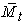: Discounted value of O&M cost

Iresv: Index of reserve capacity levelizing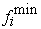: Minimum value of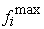: Maximum value of

Nk: Number of different types of units

PGk: Maximum capacity (MW) of a kth type unit

Nper: Number of periods considered in each year

Mt: O&M costs in year t: Heat rate at the minimum operating level (kcal/MWh) of the kth type plant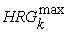: Heat rate at the maximum operating level (kcal/MWh) of the kth type plant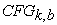: Fuel cost (\$/kcal)of the kth type plant unit located at bus b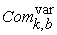: Variable O&M cost (\$/MWH) of the kth type plant unit located at bus b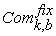: Fixed O&M cost (\$/MW) of the kth type plant unit located at bus b

Mk,b,h: Number of type-k plant units located at bus b, in period f of year t of the study period

C: The critical period as the period of the year for which the difference between the corresponding available generating capacity and the peak demand has the smallest value

Mk,st: Maintenance state, where 0 = no maintenance in time stage st (operation) and 1 = under maintenance in time stage st

Stk: Starting stage for maintenance (the week or month)

Sk: Number of stages for maintenance (the number of weeks or months)

S: ,The set of generating units involved in maintenance in the period under examination

Stk1 and Stk2: The starting times of the first and second maintenances, respectively

Vr,st: Maximum number of generating units that the maintenance crew Vr can work on simultaneously in stage st

Nu: Number of generating unit

Nst: Number of time stages in each year

G(t): Gravitational constant at time t

Mi(t): The gravitational mass related to agent i at time t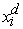: The position of ith agent in the dth dimension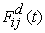: The force acting on mass ‘i’ from mass ‘j: Euclidian distance between two agents i and j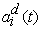: The acceleration of agent i at time t, and in direction dth: The velocity of an agent

Cr: Coefficient of cross over operator of BGA

Cm: Coefficient of mutation operator of BGA

### References

  Hossein Seifi, Mohammad Sadegh Sepasian, “Electric Power System Planning, Issues, Algorithms and Solutions”, Springer-Verlag, Berlin, 2011.In article  Shyh-Jier Huang, “Generator maintenance scheduling: a fuzzy system approach with genetic enhancement”, Electr. Power Syst. Res, 41. 233-239. 1997.In article CrossRef  João Tomé Saraiva, Marcelo Leandro Pereira, Virgílio Torrado Mendes, José Carlos Sousa, “A Simulated Annealing based approach to solve the generator maintenance scheduling problem”, Electr. Power Syst. Res, 81. 1283-1291. 2011.In article CrossRef  Y. Yare, G.K. Venayagamoorthy, “Optimal maintenance scheduling of generators using multiple swarms-MDPSO framework”, Eng Appl Artif Intel, 23. 895-910. 2010.In article CrossRef  K. Suresh, N. Kumarappan, “Particle swarm optimization based generation maintenance scheduling using probabilistic approach”, Procedia Engineering, 30. 1146-1154. 2012.In article CrossRef  Y. Yare, G.K. Venayagamoorthy, U.O. Aliyu, “Optimal generator maintenance scheduling using a modiﬁed discrete PSO”, IET Gener. Transm. Distrib, 2(6). 834-846. 2008.In article CrossRef  Keshav P. Dahal, Nopasit Chakpitak, “Generator maintenance scheduling in power systems using metaheuristic-based hybrid approaches”, Electr. Power Syst. Res, 77.771-779. 2007.In article  Ali Badri, Ahmad Norozpour Niazi, Seyyed Mehdi Hoseini, “Long Term Preventive Generation Maintenance Scheduling with Network Constraints”, Energy Procedia, 14. 1889-1895. 2012.In article CrossRef  M. Y. El-Sharkh, A.A. El-Keib, H. Chen, “A fuzzy evolutionary programming-based solution methodology for security-constrained generation maintenance scheduling”, Electr. Power Syst. Res, 67. 67-72. 2003.In article CrossRef  F. Yang, C.S. Chang, “Optimization of maintenance schedules and extents for composite power systems usingmulti-objective evolutionary algorithm”, IET Gener. Transm. Distrib, 3(10). 930-940. 2009.In article CrossRef  M.Y. El-Sharkh, A.A. El-Keib, “An evolutionary programming-based solution methodology for power generation and transmission maintenance scheduling”, Electr. Power Syst. Res, 65. 35-40. 2003.In article CrossRef  Abdolvahhab Fetanat, Gholamreza Shaﬁpour, “Generation maintenance scheduling in power systems using ant colony optimization for continuous domains based 0-1 integer programming”, Expert Syst Appl. 38. 9729-9735. 2011.In article CrossRef  Abdolvahhab Fetanat, Gholamreza Shaﬁpour, Mehdi Yavandhasani, Farrokh Ghanatir, “Optimizing Maintenance Scheduling of Generating Units in Electric Power Systems using Quantum-inspired Evolutionary Algorithm based 0-1 Integer Programming”, EUR J SCI Res, 55(2). 220-231. 2011.In article  Amir Abiri-Jahromi, Mahmud Fotuhi-Firuzabad, Masood Parvania, “Optimized Midterm Preventive Maintenance Outage Scheduling of Thermal Generating Units”, IEEE Trans. Power Syst, 27(3). 1354-1365. 2012.In article CrossRef  Grigorios A. Bakirtzis, Pandelis N. Biskas, Vasilis Chatziathanasiou, “Generation Expansion Planning by MILP considering mid-term scheduling decisions”, Electr. Power Syst. Res, 86. 98-112. 2012.In article CrossRef  C. Feng, X. Wang, F. Li, “Optimal maintenance scheduling of power producers considering unexpected unit failure”, IET Gener.Transm. Distrib, 3(5). 460-471. 2009.In article CrossRef  E. Rashedi, H. Nezamabadi-pour and S. Saryazdi, “GSA: A gravitational search algorithm”, Inform Sciences, 179(13). 2232-2248. 2009.In article CrossRef  E. Rashedi, H. Nezamabadi-pour and S. Saryazdi, “BGSA: binary gravitational search algorithm”, Natural Computation, 9(3). 1-19. 2009.In article  Y.J. Lai, C.L. Hwang, Fuzzy multiple objective decision making: methods and applications, Springer-Verlag, Berlin. 1994.In article CrossRef  I. Goroohi Sardou, M. Banejad, R. Hooshmand, A. Dastfan, “Modiﬁed shuffled frog leaping algorithm for optimal switch placement in distribution automation system using a multi-objective fuzzy approach”, IET Gener. Transm. Distrib, 6(6). 493-502. 2012.In article CrossRef  T. Niknam, H. Zeinoddini Meymand, H. Doagou Mojarrad, “A novel Multi-objective Fuzzy Adaptive Chaotic PSO algorithm for Optimal Operation Management of distribution network with regard to fuel cell power plants”, Euro. Trans. Electr. Power, 21. 1954-1983. 2011.In article CrossRef  I. Goroohi Sardou, M.T. Ameli, M.S. Sepasian and M. Ahmadian, “A Novel Genetic-based Optimization for Transmission Constrained Generation Expansion Planning”, I.J. Intelligent Systems and Applications, 1. 73-83. 2014.In article CrossRef  Allen J. Wood, Power generation, operation and control, A Wiley-Interscience Publication, University of Minnesota, 1984.In article  X.F. Wang, James McDonald, “Modern power system planning”, McGraw-Hill, London. 1994.In article  John Grainger, Jr., William Stevenson, “Power System Analysis”, McGraw-Hill. 337-338. 1994.In article  “IEEE reliability test system-96”, IEEE Trans. Power Syst, 14(3). 1010-1020.1999.In article